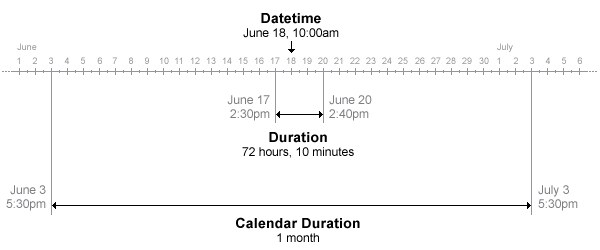# calendarDuration

## 说明## 创建对象

`M = calmonths(1:3)`
```M = 1×3 calendarDuration array 1mo 2mo 3mo ```

### 语法

``L = calendarDuration(Y,M,D)``
``L = calendarDuration(Y,M,D,H,MI,S)``
``L = calendarDuration(Y,M,D,T)``
``L = calendarDuration(X)``
``L = calendarDuration(___,'Format',displayFormat)``

### 描述

``L = calendarDuration(Y,M,D)` 根据数值数组 `Y`、`M` 和 `D`（分别包含年数、月数和天数）创建一个日历持续时间数组。`
``L = calendarDuration(Y,M,D,H,MI,S)` 还包括小时数、分钟数和秒数，分别由 `H`、`MI` 和 `S` 指定。`

``L = calendarDuration(Y,M,D,T)` 根据数值数组（包含年数、月数和天数）和持续时间数组 `T`（包含已用时间）创建一个日历持续时间数组。`
``L = calendarDuration(X)` 根据数值矩阵创建一个日历持续时间数组。`

``L = calendarDuration(___,'Format',displayFormat)` 还将 `displayFormat` 指定为 `L` 的 `Format` 属性的值。`Format` 属性更改 `L` 的显示方式，而不是更改其值。您可以将此语法与前面语法中的任何参数结合使用。`

## 属性

`y`
`q`季度（3 个月的倍数）
`m`
`w`
`d`
`t`时间（小时、分钟和秒）

## 示例

`D = datetime(2017,7,1)`
```D = datetime 01-Jul-2017 ```

`C = calmonths(0:3)`
```C = 1x4 calendarDuration 0mo 1mo 2mo 3mo ```

`M = D + C`
```M = 1x4 datetime 01-Jul-2017 01-Aug-2017 01-Sep-2017 01-Oct-2017 ```

`L = calendarDuration(1,3,15)`
```L = calendarDuration 1y 3mo 15d ```

`D = [1 3;4 2]`
```D = 2×2 1 3 4 2 ```

`T = hours([1 2; 25 12])`
```T = 2x2 duration 1 hr 2 hr 25 hr 12 hr ```

`L = calendarDuration(1,13,D,T)`
```L = 2x2 calendarDuration 2y 1mo 1d 1h 0m 0s 2y 1mo 3d 2h 0m 0s 2y 1mo 4d 25h 0m 0s 2y 1mo 2d 12h 0m 0s ```

`L = calendarDuration(1,1,5:9,'Format','mwdt')`
```L = 1x5 calendarDuration 13mo 5d 13mo 6d 13mo 1w 13mo 1w 1d 13mo 1w 2d ```

## 提示

• 有关接受或返回日历持续时间数组的函数的详细信息，请参阅日期时间

• 当您向日期时间中添加一个包含多个单位的 `calendarDuration` 数组时，MATLAB® 总是会先添加较大的单位。如果 `t` 为日期时间，则以下命令：

`t + calendarDuration(1,2,3)`
与下列语句等效：
`t + calyears(1) + calmonths(2) + caldays(3)`